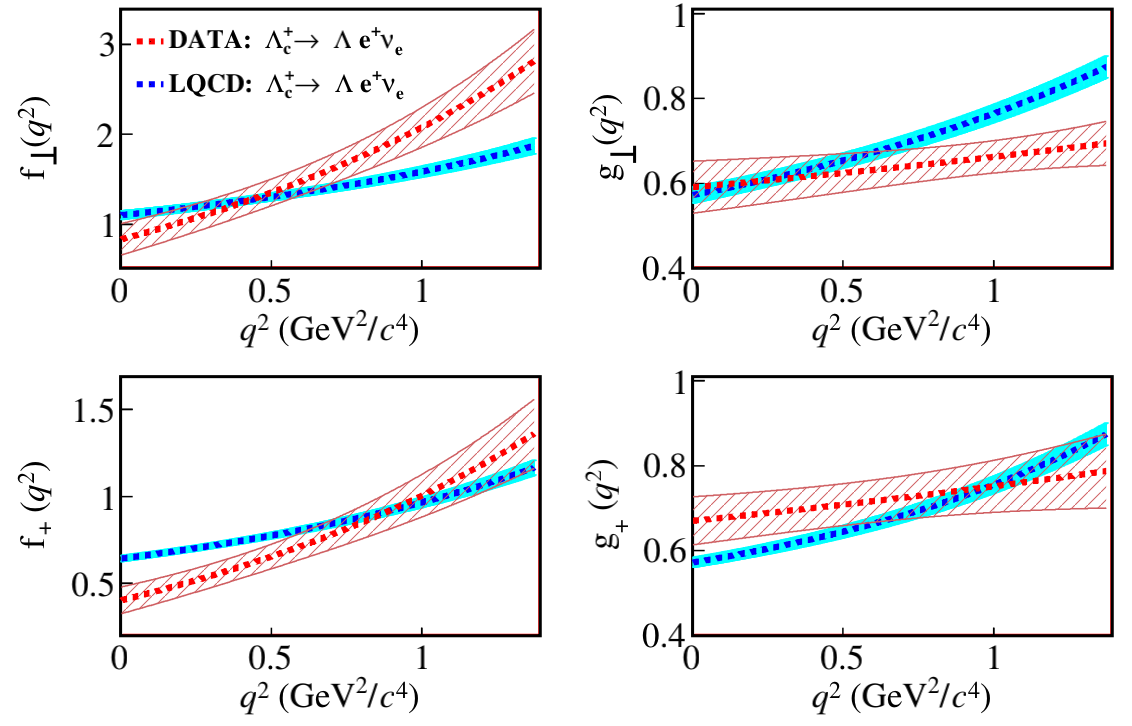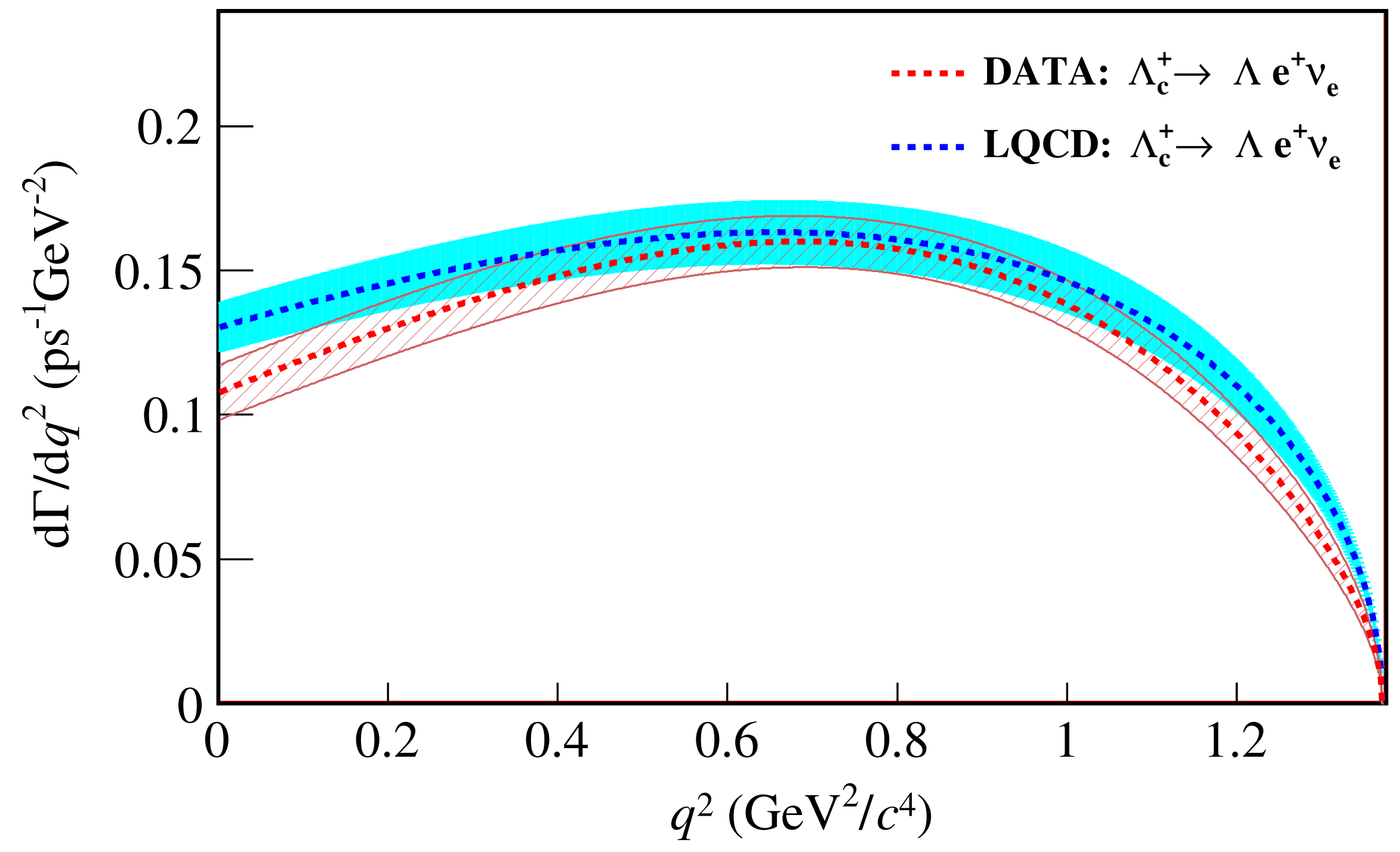DocDBHyperNewsIndicoHome

## Highlights

### Study of the Semileptonic decay Λc+ to Λe+νe

2022-12-08 Author：
PrintText Size A A

BESIII recently reported the study of the Cabibbo-favored Semileptonic (SL) decay Λc+->Λe+νe [Phys. Rev. Lett. 129, 231803 (2022)], with the largest Λc threshold data sets collected at the center-of-mass energy region between 4.6 GeV and 4.7 GeV.

The study of Λc SL decays provides valuable information about weak and strong interactions in baryons containing a heavy quark. In particular, the SL decay Λc+->Λe+νe which is the dominant component in Λc SL decays, is the most interesting to measure. Its decay rate depends on the weak quark mixing Cabibbo-Kobayashi-Maskawa (CKM) matrix element |Vcs| and strong interaction effects parameterized by form factors describing the hadronic transition between the initial and the final baryons. Measurement of |Vcs| via Λc+->Λe+νe is an important consistency test for the standard model (SM) and a probe for new physics beyond the SM. Motivated by the measurements of the absolute branching fractions (BFs) for Λc+->Λe+νe and Λc+->Λμ+νμ by BESIII experiment, the first calculation of the BFs for Λc+->Λl+νl from the lattice quantum chromodynamics (LQCD) are available in 2017. The LQCD also investigated the internal dynamics and predicted the form factors and differential decay rates in Λc+->Λe+νe decay, followed by a series of other LQCD calculations in this sector. These theoretical works are important for understanding the decay mechanism in SL decays in the charmed baryon sector. However, there is no direct experimental data for testing and calibrating these calculations.

Using the 4.5/fb Λc threshold data sets collected with BESIII detector, we report an updated measurement of the absolute BF for Λc+->Λe+νe to be (3.56±0.11±0.07)%, which improves precision of the world average value more than threefold. Combing BF of Λc+->Λe+νe measured in this work, Λc+ life time and the q2-integrated rate predicted by LQCD, we determine |Vcs|=0.936±0.017±0.025, which is in consistent with |Vcs|=0.939±0.038 measured in D->Kl+νl decays within one standard deviation. Furthermore, by analyzing the dynamics of Λc+->Λe+νe, we measure the decay form factors for the first time. Figures 1 and 2 show comparisons of the form factors and the differential decay rate between measurements in this work and that in LQCD calculations. It is noted that the dependences of measured FFs show different kinematic behavior compared to those predicted from LQCD calculations. In particular, discrepancies can be seen at high q2-region for f(q2) and g(q2), as well as at low q2-region for f+(q^2). For f+(q2) and f(q2), our measurement tends to have steeper slope than those from LQCD calculations, while on the contrary for g+(q2) and g(q2). The corresponding comparison on differential decay rates, shown in Figure 2, gives fair agreement throughout the q2 region.Figure 1. Comparison of form factors with LQCD calculations.Figure 2. Comparison of the differential decay rates with LQCD predictions.